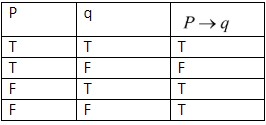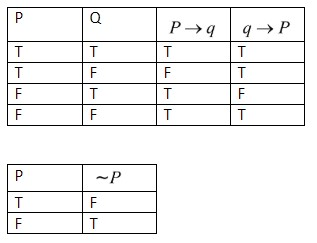QuestionAnswers

# If the truth value of p is T, q is F, then truth values of ($p\to q$) and $\left( q\to p \right)V\left( \nu p \right)$ are respectively.a.F, Fb.F, Tc.T, Fd.T, THint: In $pVq$, V represents a non-exclusive or i.e. $pVq$ is true when any of p, q is true and also when both are true. Draw the table of logical implication. Find the truth value of ($p\to q$), ($q\to p$) and ($\nu p$).

Substituting all the values, find the truth value.
The truth or falsehood of a proposition is called its truth value. In $P\nu q,$V represents a non-exclusive or i.e. $p\nu q$ is true when any of p, q is true and also when both are true.
Here, ($p\to q$) implies that,
‘p’ implies ‘q’ means that if p is true, then q must also be true.
The true statement “p implies q” is also written “if p then q” or sometimes “q if p”. Statement p is called the premise of the implication and q is called the conclusion.
For eg: -$P\to Q$ is logically equivalent to $P\nu Q$. “If a number is a multiple of 4, then it is even” is equivalent to a “number is not a multiple of 4 or (else) it is even”.
Now in the question it is given that the true value of p is T and the true value of q is F.
We need to find the value of ($p\to q$).
From the table of logical implication,$\therefore P\to q$ when P is T and q is F.
The 2nd case,
$\therefore P\to q$ is F.
Similarly, to find $q\to P$.From the above table for the 2nd case, where the truth value of P is T and truth value of Q is F.
$P\to q$is F, $q\to p$is T and $\sim p=F$
We need to find $\left( q\to p \right)V\left( \sim p \right)=F$.
i.e. if T V T comes then the truth value is T.
if T V F, then the truth value is F.
if F V F, then the truth value is F.
if F V T, then the truth value is F.
Hence, the correct answer is (a),

Note: You should remember or be able to construct the truth tables for the logical connectives. You will use these tables to construct tables for more complicated sentences. It’s easier to demonstrate what to do than to describe it in words. When you construct a truth table, you will have to consider all possible assignments of True (T) and False (F) to the component statement.

View Notes
What is the full form of phd?What is the full form of CFTWhat is the Cell Envelope?What is the Placebo EffectWhat is Syphilis?Where There is a Will There is a Way EssayThe Difference Between an Animal that is A Regulator and One that is A ConformerWhat is Polio?What is the Difference Between Trade and Commerce?CBSE Class 10 Maths Question Paper 2017CBSE Class 10 Maths Question Paper 2020Previous Year Question Paper of CBSE Class 10 EnglishMaths Question Paper for CBSE Class 10 - 2011Maths Question Paper for CBSE Class 10 - 2008Maths Question Paper for CBSE Class 10 - 2012Maths Question Paper for CBSE Class 10 - 2009Maths Question Paper for CBSE Class 10 (2016)Maths Question Paper for CBSE Class 10 - 2010Maths Question Paper for CBSE Class 10 - 2007NCERT Solutions for Class 11 Maths Chapter 14RS Aggarwal Class 10 Solutions - T-Ratios of Some Particular AnglesNCERT Solutions Class 11 English Woven Words Poem Chapter 5 The World is too Much With UsNCERT Exemplar for Class 9 Science Chapter 2 - Is Matter Around Us Pure (Book Solutions)Lakhmir Singh Chemistry Class 9 Solutions Chapter 2 - Is Matter Around Us PureNCERT Solutions Class 11 English Woven Words Essay Chapter 5 What is a Good BookRD Sharma Class 12 Maths Solutions Chapter 15 - Mean Value TheoremsNCERT Solutions for Class 9 Science Chapter 2 Is Matter Around Us Pure in HindiNCERT Solutions for Class 9 English Moments Chapter 8 - A House is Not a HomeNCERT Solutions for Class 12 Chemistry